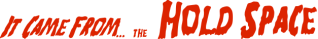Next16

• Strategy:

• Let \$held be the previously read line

• Read lines one at a time

• If the current line is an underline, print uc \$held and discard it

• Otherwise, print \$held and store the current line in \$held

• At the end of the file, print \$held if there is one

• In one of the "P" languages, this is not too hard:

```        #!/usr/bin/perl

while (<>) {
if (/^(.)\1+\$/ && defined(\$held) && length(\$_) == length(\$held)) {
# It's an underline
print uc(\$held);
undef \$held;
} else {
print \$held if defined \$held;
\$held = \$_;
}
}
print \$held if defined \$held;
```
• Not the loveliest code, but it is fairly straightforward